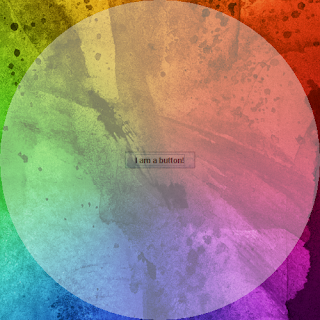# Create Shaped Transparent JFrames in Java Swing

Here is a sample tutorial making fun with JFrame, giving it all the shapes that you can to a frame. Make a frame look circular, ellipse, curve, rounded rectanble, some other different shape that cannot be defined and may not be seen. Here i will show how you can customize your JFrame as you wish.Circular ShapeCubic Curve Shape

import javax.swing.*;
import java.awt.*;
import java.awt.event.*;
import java.awt.geom.*;
class ShapedJFrame extends JFrame
{
JButton b1;
public ShapedJFrame()
{
setTitle("Transparent JFrame Demo");
setLayout(new GridBagLayout());
setDefaultCloseOperation(EXIT_ON_CLOSE);
setUndecorated(true);
setVisible(true);
setOpacity(0.4f);

// Try different shapes

// Normal rounded rectangle
//setShape(new RoundRectangle2D.Double(0,0,getWidth(),getHeight(),20,40));

//Ellipse shape
//setShape(new Ellipse2D.Double(0,0,400,400));

// Cubic Curve. Looks funny:)
//setShape(new CubicCurve2D.Double(0,0,500,5,200,0,500,500));

// For three-fourth circle
//setShape(new Arc2D.Double(new Rectangle2D.Double(0,0,500,500),90,270,Arc2D.PIE));

// For round circle shape
setShape(new Arc2D.Double(new Rectangle2D.Double(0,0,500,500),90,360,Arc2D.PIE));

b1=new JButton("I am a button!");

setSize(500,500);
setLocationRelativeTo(null);
}
public static void main(String args[])
{
new ShapedJFrame();
}
}
Round Rectangle:  Mostly found. A Rectangle with rounded corners. Double is a static class in the RoundRectangle2D class located in the java.awt.geom package.
new RoundRectangle2D.Double(double x, double y, double width, double height,double cornerwidth,double cornerheight)
QuadCurve.Double(double x1,double y1,double ctrlx,double ctrly,double x2,double y2):  Another shape.

CubicCurve2D.Double(double x1,double y1, double ctrlx1,double ctrly1,double ctrlx2,double ctrly2,double x2,double y2): The cubic curve shape. Define it yourself seeing what shape you get :)

Arc2D.Double(Rectangle2D rd,double start,double end, int type): Rectangle2D is an abstract class therefore, we use Rectangle2D.Double class which is static inner class. In this we specify, the x,y coordinates and width and height. Next the type of the arc. Possible types are Arc2D.CHORD, Arc2D.PIE, Arc2D.OPEN.